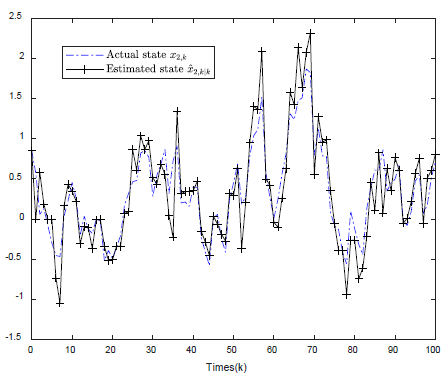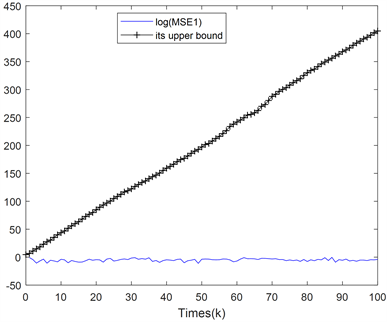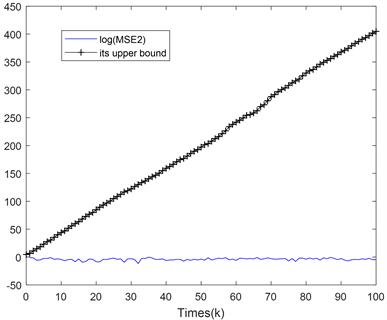# 带有随机发生非线性的时变动态系统非脆弱递归状态估计Nonfragile Recursive State Estimation for Time-Varying Dynamic Systems with Random Nonlinearity

DOI: 10.12677/AAM.2019.81008, PDF, HTML, XML, 下载: 429  浏览: 1,826

Abstract: A class of time-varying dynamic systems with random nonlinearity are studied in this paper. This system contains the following network phenomena: The system’s uncertainty, random occurrence non-linearity and non-fragility, among which random occurrence non-linearity is represented by a random variable obeying Bernoulli distribution, non-fragility satisfies the norm bounded uncer-tainty. The aim of this paper is to propose a robust non-fragile state estimation algorithm for sto-chastic nonlinearity. Since the estimated error covariance matrix cannot be expressed accurately, we find the upper bound of the error covariance matrix and minimize the trace of the upper bound by designing the filter gain matrix. Finally, a numerical simulation is given to illustrate the practi-cability of the algorithm.

1. 引言

2. 主要结论

${x}_{k+1}=\left({A}_{k}+{\xi }_{k}{\stackrel{¯}{A}}_{k}\right){x}_{k}+{\alpha }_{k}f\left({x}_{k}\right)+{B}_{k}{\omega }_{k}$

${y}_{k}=h\left({x}_{k}\right)+{v}_{k}$ (1)

$\text{Prob}\left\{{\alpha }_{k}=1\right\}=\mathbb{E}\left\{{\alpha }_{k}\right\}={\stackrel{¯}{\alpha }}_{k}$

$\text{Prob}\left\{{\xi }_{k}=1\right\}=\mathbb{E}\left\{{\xi }_{k}\right\}={\stackrel{¯}{\xi }}_{k}$ (2)

$‖f\left({x}_{k}\right)-f\left({z}_{k}\right)‖\le l‖{x}_{k}-{z}_{k}‖$ (3)

$‖h\left({x}_{k}\right)-h\left({z}_{k}\right)‖\le l‖{x}_{k}-{z}_{k}‖$

${\stackrel{^}{x}}_{k+1|k}=\left({A}_{k}+{\stackrel{¯}{\xi }}_{k}{\stackrel{¯}{A}}_{k}\right){\stackrel{^}{x}}_{k|k}+{\stackrel{¯}{\alpha }}_{k}f\left({\stackrel{^}{x}}_{k}\right)$ (4)

${\stackrel{^}{x}}_{k+1|k+1}={\stackrel{^}{x}}_{k+1|k}+\left({K}_{k+1}+\Delta {K}_{k+1}\right)\left[{y}_{k+1}-h\left({\stackrel{^}{x}}_{k+1|k}\right)\right]$

$E\left\{\left({x}_{k+1}-{\stackrel{^}{x}}_{k+1|k+1}\right){\left({x}_{k+1}-{\stackrel{^}{x}}_{k+1|k+1}\right)}^{\text{T}}\right\}\le {\sum }_{k+1|k+1}$ (5)

3. 具有随机发生非线性的递推滤波器设计

$a{b}^{\text{T}}+b{a}^{\text{T}}\le \epsilon a{a}^{\text{T}}+{\epsilon }^{-1}b{b}^{\text{T}}$ (6)

$\begin{array}{l}\left(A+HFE\right)X{\left(A+HFE\right)}^{\text{T}}\\ \le A{\left({X}^{-1}-\gamma {E}^{\text{T}}E\right)}^{-1}{A}^{\text{T}}+{\gamma }^{-1}H{H}^{\text{T}}\end{array}$ (7)

${\stackrel{˜}{x}}_{k+1|k}={x}_{k+1}-{\stackrel{^}{x}}_{k+1|k}$ ，为一步预测误差，

${\stackrel{˜}{x}}_{k+1|k+1}={x}_{k+1}-{\stackrel{^}{x}}_{k+1|k+1}$ ，为滤波误差，

$\begin{array}{c}{\stackrel{˜}{x}}_{k+1|k}={A}_{k}{\stackrel{˜}{x}}_{k|k}+{\stackrel{¯}{\zeta }}_{k}{\stackrel{¯}{A}}_{k}{\stackrel{˜}{x}}_{k|k}+{\stackrel{¯}{\zeta }}_{k}{\stackrel{¯}{A}}_{k}{x}_{k}+{\stackrel{˜}{\alpha }}_{k}f\left({x}_{k}\right)\\ \text{\hspace{0.17em}}\text{\hspace{0.17em}}+{\stackrel{¯}{\alpha }}_{k}\left[f\left({x}_{k}\right)-f\left({\stackrel{^}{x}}_{k}\right)\right]+{B}_{k}{\omega }_{k}\end{array}$ (8)

$\begin{array}{c}{\stackrel{˜}{x}}_{k+1|k+1}={x}_{k+1}-{\stackrel{^}{x}}_{k+1|k+1}\\ ={\stackrel{˜}{x}}_{k+1|k}-\left({K}_{k+1}+\Delta {K}_{k+1}\right)\left[h\left({x}_{k+1}\right)+{v}_{k+1}-h\left({\stackrel{^}{x}}_{k+1|k}\right)\right]\\ ={\stackrel{˜}{x}}_{k+1|k}-\left({K}_{k+1}+\Delta {K}_{k+1}\right)\left[h\left({x}_{k+1}\right)-h\left({\stackrel{^}{x}}_{k+1|k}\right)\right]\\ \text{}-\left({K}_{k+1}+\Delta {K}_{k+1}\right){v}_{k+1}\end{array}$ (9)

${X}_{k+1}=E\left\{{x}_{k+1}{x}_{k+}^{\text{T}}{}_{1}\right\}$ 表达式如下

$\begin{array}{c}{X}_{k+1}\le \left(1+{\epsilon }_{1}+{\epsilon }_{2}\right){A}_{k}{X}_{k}{A}_{k}^{Τ}+\left(1+{\epsilon }_{1}^{-1}+{\epsilon }_{2}\right){\stackrel{¯}{\xi }}_{k}{\stackrel{¯}{A}}_{k}{X}_{k}{\stackrel{¯}{A}}^{Τ}{}_{k}\\ \text{}+\left(1+{\epsilon }_{2}^{-1}+{\epsilon }_{3}^{-1}\right){\stackrel{¯}{\alpha }}_{k}{l}^{2}tr\left({X}_{k}\right)I+{B}_{k}{Q}_{k}{B}_{k}^{Τ}\\ \text{:}={\stackrel{¯}{X}}_{k+1}\end{array}$ (10)

$\begin{array}{c}{X}_{k+1}=E\left\{{x}_{k+1}{x}_{k+1}^{\text{T}}\right\}\\ ={A}_{k}{X}_{k}{A}_{k}^{\text{T}}+\stackrel{¯}{\xi }{A}_{k}{X}_{k}{\stackrel{¯}{A}}_{k}^{\text{T}}+{\stackrel{¯}{\alpha }}_{k}E\left\{f\left({x}_{k}\right){f}^{\text{T}}\left({x}_{k}\right)\right\}\\ \text{\hspace{0.17em}}\text{\hspace{0.17em}}+{B}_{k}Q{B}_{k}^{\text{T}}+{H}_{1}+{H}_{1}^{\text{T}}+{H}_{2}+{H}_{2}^{\text{T}}+{H}_{3}+{H}_{3}^{\text{T}}\end{array}$

$\begin{array}{l}{H}_{1}={\xi }_{k}{A}_{k}{X}_{k}{\stackrel{¯}{A}}^{Τ}{}_{k}\\ {H}_{2}={\alpha }_{k}{A}_{k}E\left\{{x}_{k}{f}^{Τ}\left({x}_{k}\right)\right\}\\ {H}_{3}={\xi }_{k}{\partial }_{k}{\stackrel{¯}{A}}_{k}E\left\{{x}_{k}{f}^{Τ}\left({x}_{k}\right)\right\}\end{array}$ (11)

$\begin{array}{l}{H}_{1}+{H}_{1}^{Τ}\le {\epsilon }_{1}{A}_{k}{X}_{k}{A}_{k}^{Τ}+{\epsilon }_{1}^{-1}{\stackrel{¯}{\xi }}_{k}{\stackrel{¯}{A}}_{k}{X}_{k}{\stackrel{¯}{A}}^{Τ}{}_{k}\\ {H}_{2}+{H}_{2}^{Τ}\le {\epsilon }_{2}{A}_{k}{X}_{k}{A}_{k}^{Τ}+{\epsilon }_{2}^{-1}{\stackrel{¯}{\alpha }}_{k}E\left\{f\left({x}_{k}\right){f}^{Τ}\left({x}_{k}\right)\right\}\\ {H}_{3}+{H}_{3}^{Τ}\le {\epsilon }_{3}{\stackrel{¯}{\xi }}_{k}{\stackrel{¯}{A}}_{k}{X}_{k}{\stackrel{¯}{A}}^{Τ}{}_{k}+{\epsilon }_{3}^{-1}{\stackrel{¯}{\alpha }}_{k}E\left\{f\left({x}_{k}\right){f}^{Τ}\left({x}_{k}\right)\right\}\end{array}$

$\begin{array}{c}{X}_{k+1}\le \left(1+{\epsilon }_{1}+{\epsilon }_{2}\right){A}_{k}{X}_{k}{A}_{k}^{Τ}+\left(1+{\epsilon }_{1}^{-1}+{\epsilon }_{2}\right){\stackrel{¯}{\xi }}_{k}{\stackrel{¯}{A}}_{k}{X}_{k}{\stackrel{¯}{A}}^{Τ}{}_{k}\\ \text{\hspace{0.17em}}\text{\hspace{0.17em}}+\left(1+{\epsilon }_{2}^{-1}+{\epsilon }_{3}^{-1}\right){\stackrel{¯}{\alpha }}_{k}E\left\{f\left({x}_{k}\right){f}^{Τ}\left({x}_{k}\right)\right\}+{B}_{k}{Q}_{k}{B}_{k}^{Τ}\end{array}$ (12)

$f\left({x}_{k}\right){f}^{Τ}\left({x}_{k}\right)\le {‖f\left({x}_{k}\right)‖}^{2}I=f\left({x}_{k}\right){f}^{Τ}\left({x}_{k}\right)I$

$E\left\{f\left({x}_{k}\right){f}^{Τ}\left({x}_{k}\right)\right\}\le {l}^{2}E\left\{{x}_{k}{x}_{k}^{Τ}\right\}={l}^{2}tr\left({X}_{k}\right)$ (13)

$\begin{array}{c}{X}_{k+1}\le \left(1+{\epsilon }_{1}+{\epsilon }_{2}\right){A}_{k}{X}_{k}{A}_{k}^{Τ}+\left(1+{\epsilon }_{1}^{-1}+{\epsilon }_{2}\right){\stackrel{¯}{\xi }}_{k}{\stackrel{¯}{A}}_{k}{X}_{k}{\stackrel{¯}{A}}^{Τ}{}_{k}\\ \text{\hspace{0.17em}}\text{\hspace{0.17em}}+\left(1+{\epsilon }_{2}^{-1}+{\epsilon }_{3}^{-1}\right){\stackrel{¯}{\alpha }}_{k}{l}^{2}tr\left({X}_{k}\right)I+{B}_{k}{Q}_{k}{B}_{k}^{Τ}\end{array}$ (14)

$\begin{array}{c}{P}_{k+1|k}={A}_{k}{P}_{k|k}{A}_{k}^{Τ}+{\stackrel{¯}{\xi }}_{k}^{2}{\stackrel{¯}{A}}_{k}{P}_{k|k}{\stackrel{¯}{A}}^{Τ}{}_{k}+{\stackrel{¯}{\xi }}^{2}{}_{k}\left(1-{\stackrel{¯}{\xi }}_{k}\right){\stackrel{¯}{A}}_{k}E\left\{{x}_{k}{x}_{k}^{Τ}\right\}{\stackrel{¯}{A}}^{Τ}\\ \text{}+{\stackrel{¯}{\alpha }}_{k}\left(1-{\stackrel{¯}{\alpha }}_{k}\right)E\left\{f\left({x}_{k}\right){f}^{Τ}\left({x}_{k}\right)\right\}\\ \text{}+{\stackrel{¯}{\alpha }}_{k}^{2}E\left\{\left[f\left({x}_{k}\right)-f\left({\stackrel{^}{x}}_{k}\right)\right]{\left[f\left({x}_{k}\right)-f\left({\stackrel{^}{x}}_{k}\right)\right]}^{Τ}\right\}\\ \text{}+{B}_{k}{Q}_{k}{B}_{k}^{Τ}+L+{L}^{Τ}\end{array}$ (15)

$L=E\left\{\left({A}_{k}{\stackrel{˜}{x}}_{k|k}+{\stackrel{¯}{\xi }}_{k}{\stackrel{¯}{A}}_{k}{\stackrel{˜}{x}}_{k|k}\right){\stackrel{¯}{\alpha }}_{k}{\left[f\left({x}_{k}\right)-f\left({\stackrel{^}{x}}_{k}\right)\right]}^{Τ}\right\}$ ${P}_{k|k}=E\left\{{\stackrel{˜}{x}}_{k|k}{\stackrel{˜}{x}}^{\text{T}}{}_{k|k}\right\}$ 为滤波误差协方差。

$\begin{array}{c}{P}_{k+1|k+1}=E\left\{{\stackrel{˜}{x}}_{k+1\text{|}k+1}{\stackrel{˜}{x}}_{k+1|k+1}^{Τ}\right\}\\ ={P}_{k+1|k}+\left({K}_{k+1}+\Delta {K}_{k+1}\right)E\left\{\left[h\left({x}_{k+1}\right)-h\left({\stackrel{^}{x}}_{k+1|k}\right)\right]\\ \text{\hspace{0.17em}}\text{\hspace{0.17em}}×{\left[h\left({x}_{k+1}\right)-h\left({\stackrel{^}{x}}_{k+1|k}\right)\right]}^{Τ}\right\}×{\left({K}_{k+1}+\Delta {K}_{k+1}\right)}^{Τ}\\ \text{\hspace{0.17em}}\text{\hspace{0.17em}}+\left({K}_{k+1}+\Delta {K}_{k+1}\right){R}_{k+1}{\left({K}_{k+1}+\Delta {K}_{k+1}\right)}^{Τ}+G+{G}^{Τ}\end{array}$ (16)

$G=E\left\{{\stackrel{˜}{x}}_{k+1|k}{\left[h\left({x}_{k+1}\right)-h\left({\stackrel{^}{x}}_{k+1|k}\right)\right]}^{Τ}{\left({K}_{k+1}+\Delta {K}_{k+1}\right)}^{Τ}\right\}$

$\begin{array}{c}{\Sigma }_{k+1|k}=\left(1+{\eta }_{1}\right)\left({A}_{k}+{\stackrel{¯}{\xi }}_{k}{\stackrel{¯}{A}}_{k}\right){\Sigma }_{k|k}{\left({A}_{k}+{\stackrel{¯}{\xi }}_{k}{\stackrel{¯}{A}}_{k}\right)}^{Τ}\\ \text{\hspace{0.17em}}\text{\hspace{0.17em}}+{\stackrel{¯}{\xi }}_{k}\left(1-{\stackrel{¯}{\xi }}_{k}\right){\stackrel{¯}{A}}_{k}{X}_{k+1}{\stackrel{¯}{A}}_{k}^{Τ}+{\stackrel{¯}{\alpha }}_{k}\left(1-{\stackrel{¯}{\alpha }}_{k}\right){l}^{2}tr\left({\stackrel{¯}{X}}_{k}\right)I\\ \text{\hspace{0.17em}}\text{\hspace{0.17em}}+\left(1+{\eta }_{1}^{-1}\right){\stackrel{¯}{\alpha }}_{k}^{2}{l}^{2}tr\left({\Sigma }_{k|k}\right)I+{B}_{k}{Q}_{k}{B}_{k}^{Τ}\end{array}$

$\begin{array}{c}{\Sigma }_{k+1|k+1}=\left(1+{\eta }_{2}\right){\Sigma }_{k+1|k}+\left(1+{\eta }_{2}^{-1}\right){l}^{2}tr\left({\Sigma }_{k+1|k}\right)\\ \text{\hspace{0.17em}}\text{\hspace{0.17em}}×\left[{K}_{k+1}{\left(I-{\gamma }_{1}{N}_{k+1}^{Τ}{N}_{k+1}\right)}^{-1}{K}_{k+1}^{Τ}+{\gamma }_{1}^{-1}{M}_{k+1}{M}_{k+1}^{Τ}\right]\\ \text{\hspace{0.17em}}\text{\hspace{0.17em}}+{K}_{k+1}{\left({R}_{k+1}^{-1}-{\gamma }_{2}{N}_{k+1}^{Τ}{N}_{k+1}\right)}^{-1}{K}_{k+1}^{Τ}+{\gamma }_{2}^{-1}{M}_{k+1}{M}_{k+1}^{Τ}\end{array}$ (17)

${\sum }_{0|0}={P}_{0|0}>0$ 有正解 ${\sum }_{k+1|k}$${\sum }_{k+1|k+1}$ ，并满足以下两个约束条件：

${\gamma }_{1}^{-1}I-{N}_{k+1}{N}_{k+1}^{Τ}>0$

${\gamma }_{2}^{-1}I-{N}_{k+1}{R}_{k+1}{N}_{k+1}^{Τ}>0$

${K}_{k+1}=\left(1+{\eta }_{2}^{-1}\right){l}^{2}tr\left({\sum }_{k+1|k}\right){\left\{{\left(I-{\gamma }_{1}{N}_{k+1}^{Τ}{N}_{k+1}\right)}^{-1}+{\left({R}_{k+1}^{-1}-{\gamma }_{2}{N}_{k+1}^{Τ}{N}_{k+1}\right)}^{-1}\right\}}^{-1}$ (18)

$\begin{array}{c}L+{L}^{Τ}\le {\eta }_{1}\left({A}_{k}{P}_{k|k}{A}_{k}^{Τ}+{\xi }_{k}^{2}{\stackrel{¯}{A}}_{k}{P}_{k|k}{\stackrel{¯}{A}}^{Τ}{}_{k}\right)\\ \text{\hspace{0.17em}}\text{\hspace{0.17em}}+{\eta }_{1}^{-1}{\stackrel{¯}{\alpha }}_{k}^{2}E\left\{\left[f\left({x}_{k}\right)-f\left({\stackrel{^}{x}}_{k}\right)\right]{\left[f\left({x}_{k}\right)-f\left({\stackrel{^}{x}}_{k}\right)\right]}^{Τ}\right\}\end{array}$ (19)

$\begin{array}{c}{P}_{k+1|k}\le \left(1+{\eta }_{1}\right)\left({A}_{k}+{\stackrel{¯}{\xi }}_{k}{\stackrel{¯}{A}}_{k}\right){P}_{k|k}{\left({A}_{k}+{\stackrel{¯}{\xi }}_{k}{\stackrel{¯}{A}}_{k}\right)}^{Τ}\\ \text{\hspace{0.17em}}\text{\hspace{0.17em}}+{\stackrel{¯}{\xi }}_{k}\left(1-{\stackrel{¯}{\xi }}_{k}\right){\stackrel{¯}{A}}_{k}E\left\{{x}_{k}{x}_{k}^{Τ}\right\}{\stackrel{¯}{A}}_{k}^{Τ}+{\stackrel{¯}{\alpha }}_{k}\left(1-{\stackrel{¯}{\alpha }}_{k}\right)E\left\{f\left({x}_{k}\right){f}^{Τ}\left({x}_{k}\right)\right\}\\ \text{\hspace{0.17em}}\text{\hspace{0.17em}}+\left(1+{\eta }_{1}^{-1}\right){\stackrel{¯}{\alpha }}_{k}^{2}E\left\{\left[f\left({x}_{k}\right)-f\left({\stackrel{^}{x}}_{k}\right)\right]×{\left[f\left({x}_{k}\right)-f\left({\stackrel{^}{x}}_{k}\right)\right]}^{Τ}\right\}+{B}_{k}{Q}_{k}{B}_{k}^{Τ}\end{array}$ (20)

$\begin{array}{l}\left(f\left({x}_{k}\right)-f\left({\stackrel{^}{x}}_{k}\right)\right){\left(f\left({x}_{k}\right)-f\left({\stackrel{^}{x}}_{k}\right)\right)}^{Τ}\\ \le {‖f\left({x}_{k}\right)-f\left({\stackrel{^}{x}}_{k}\right)‖}^{2}I\\ =f\left({x}_{k}\right)-f{\left({\stackrel{^}{x}}_{k}\right)}^{Τ}f\left({x}_{k}\right)-f\left({\stackrel{^}{x}}_{k}\right)I\end{array}$ (21)

$\begin{array}{l}E\left\{{\left[f\left({x}_{k}\right)-f\left({\stackrel{^}{x}}_{k}\right)\right]}^{Τ}\left[f\left({x}_{k}\right)-f\left({\stackrel{^}{x}}_{k}\right)\right]\right\}\\ \le {l}^{2}E\left\{{\stackrel{˜}{x}}_{k}^{Τ}{\stackrel{˜}{x}}_{k}\right\}={l}^{2}tr\left({P}_{k|k}\right)\end{array}$ (22)

$\begin{array}{c}{P}_{k+1|k}\le \left(1+{\eta }_{1}\right)\left({A}_{k}+{\stackrel{¯}{\xi }}_{k}{\stackrel{¯}{A}}_{k}\right){P}_{k|k}{\left({A}_{k}+{\stackrel{¯}{\xi }}_{k}{\stackrel{¯}{A}}_{k}\right)}^{Τ}\\ \text{\hspace{0.17em}}\text{\hspace{0.17em}}+{\stackrel{¯}{\xi }}_{k}\left(1-{\stackrel{¯}{\xi }}_{k}\right){\stackrel{¯}{A}}_{k}{X}_{k+1}{\stackrel{¯}{A}}_{k}^{Τ}+{\stackrel{¯}{\alpha }}_{k}\left(1-{\stackrel{¯}{\alpha }}_{k}\right){l}^{2}tr\left({X}_{k}\right)I\\ \text{\hspace{0.17em}}\text{\hspace{0.17em}}+\left(1+{\eta }_{1}^{-1}\right){\stackrel{¯}{\alpha }}_{k}^{2}{l}^{2}tr\left({P}_{k|k}\right)I+{B}_{k}{Q}_{k}{B}_{k}^{Τ}\end{array}$

$\begin{array}{c}G+{G}^{\text{T}}\le {\eta }_{2}{P}_{k+1|k}+{\eta }_{2}^{-1}\left({K}_{k+1}+\Delta {K}_{k+1}\right)×E\left\{\left[h\left({x}_{k+1}\right)-h\left({\stackrel{^}{x}}_{k+1|k}\right)\right]\\ \text{\hspace{0.17em}}\text{\hspace{0.17em}}×{\left[h\left({x}_{k+1}\right)-h\left({\stackrel{^}{x}}_{k+1}\right)\right]}^{\text{T}}\right\}{\left({K}_{k+1}+\Delta {K}_{k+1}\right)}^{\text{T}}\end{array}$ (23)

$\begin{array}{c}{P}_{k+1|k+1}\le \left(1+{\eta }_{2}\right){P}_{k+1|k}+\left(1+{\eta }_{2}^{-1}\right)\left({K}_{k+1}+\Delta {K}_{k+1}\right)\\ \text{\hspace{0.17em}}\text{\hspace{0.17em}}×E\left\{\left[h\left({x}_{k+1}\right)-h\left({\stackrel{^}{x}}_{k+1}\right)\right]{\left[h\left({x}_{k+1}\right)-h\left({\stackrel{^}{x}}_{k+1}\right)\right]}^{Τ}\right\}\\ \text{\hspace{0.17em}}\text{\hspace{0.17em}}×{\left({K}_{k+1}+\Delta {K}_{k+1}\right)}^{Τ}+\left({K}_{k+1}+\Delta {K}_{k+1}\right){R}_{k+1}{\left({K}_{k+1}+\Delta {K}_{k+1}\right)}^{Τ}\end{array}$ (24)

$\begin{array}{l}\left({K}_{k+1}+\Delta {K}_{k+1}\right)E\left\{\left[h\left({x}_{k+1}\right)-h\left({\stackrel{^}{x}}_{k+1}\right)\right]\\ \text{ }×\left[h\left({x}_{k+1}\right)-h{\left({\stackrel{^}{x}}_{k+1}\right)}^{Τ}\right]\right\}{\left({K}_{k+1}+\Delta {K}_{k+1}\right)}^{Τ}\\ \le {l}^{2}tr\left({P}_{k|k}\right)\left({K}_{k+1}+\Delta {K}_{k+1}\right){\left({K}_{k+1}+\Delta {K}_{k+1}\right)}^{Τ}\\ \le {l}^{2}tr\left({P}_{k+1|k}\right)\left[{K}_{k+1}{\left(I-{\gamma }_{1}{N}_{k+1}^{Τ}N\right)}^{-1}{K}_{k+1}^{Τ}+{\gamma }_{1}^{-1}{M}_{k+1}{M}_{k+1}^{Τ}\right]\end{array}$ (25)

$\begin{array}{l}\left({K}_{k+1}+\Delta {K}_{k+1}\right){R}_{k+1}{\left({K}_{k+1}+\Delta {K}_{k+1}\right)}^{Τ}\\ \le {K}_{k+1}{\left({R}_{k+1}^{-1}-{\gamma }_{2}{N}_{k+1}^{Τ}{N}_{k+1}\right)}^{-1}{K}_{k+1}^{Τ}+{\gamma }_{2}^{-1}{M}_{k+1}{M}_{k+1}^{Τ}\end{array}$ (26)

$\begin{array}{c}{P}_{k+1|k+1}\le \left(1+{\eta }_{2}\right){P}_{k+1|k}+\left(1+{\eta }_{2}^{-1}\right){l}^{2}tr\left({P}_{k+1|k}\right)\\ \text{\hspace{0.17em}}\text{\hspace{0.17em}}×\left[{K}_{k+1}{\left(I-{\gamma }_{1}{N}_{k+1}^{Τ}{N}_{k+1}\right)}^{-1}{K}_{k+1}^{Τ}+{\gamma }_{1}^{-1}{M}_{k+1}{M}_{k+1}^{Τ}\right]\\ \text{\hspace{0.17em}}\text{\hspace{0.17em}}+{K}_{k+1}{\left({R}_{k+1}^{-1}-{\gamma }_{2}{N}_{k+1}^{Τ}{N}_{k+1}\right)}^{-1}{K}_{k+1}^{Τ}+{\gamma }_{2}^{-1}{M}_{k+1}{M}_{k+1}^{Τ}\end{array}$ (27)

${K}_{k+1}=\left(1+{\eta }_{2}^{-1}\right){l}^{2}tr\left({\sum }_{k+1|k}\right){\left\{{\left(I-{\gamma }_{1}{N}_{k+1}^{Τ}{N}_{k+1}\right)}^{-1}+{\left({R}_{k+1}^{-1}-{\gamma }_{2}{N}_{k+1}^{Τ}{N}_{k+1}\right)}^{-1}\right\}}^{-1}$ (28)

4. 数值仿真

${A}_{k}=\left[\begin{array}{cc}-0.8& 0.6\\ -0.9& 0.3\end{array}\right]$ , ${\stackrel{¯}{A}}_{k}=\left[\begin{array}{cc}-1.6& 1.2\\ 1.4& 0.8\end{array}\right]$ ,

${B}_{k}={\left[\begin{array}{cc}0.60& 0.60\end{array}\right]}^{\text{T}}$ , ${M}_{k}=\left[\begin{array}{cc}-0.4& 0\\ 0& 0.4\end{array}\right]$ ,

${N}_{k}=\left[\begin{array}{cc}0.3& 0\\ 0& 0.3\end{array}\right]$ ,

$f\left({x}_{k}\right)={\left[\mathrm{sin}{x}_{1,k}\mathrm{cos}{x}_{2,k}\text{\hspace{0.17em}}\text{\hspace{0.17em}}\text{\hspace{0.17em}}\mathrm{cos}{x}_{1,k}\right]}^{\text{T}}$ ,

$h\left({x}_{k}\right)={\left[\mathrm{cos}{x}_{2,k}\text{\hspace{0.17em}}\mathrm{sin}{x}_{1,k}\text{\hspace{0.17em}}\text{\hspace{0.17em}}{\mathrm{sin}}^{2}{x}_{2,k}\right]}^{\text{T}}$ ,

${\gamma }_{1,k+1}=1.5$ ,

${\gamma }_{2,k+1}=1.2$ ,

${\epsilon }_{i}=1\left(i=1,2,3\right),{\eta }_{1}={\eta }_{2}=1,{Q}_{k}=0.1$

${R}_{k}=diag\left\{0.1,0.1\right\},{\stackrel{¯}{\alpha }}_{k}=0.76,\stackrel{¯}{\xi }=0.65$ ,

$l=3$ ;

${x}_{0|0}={\left[\begin{array}{cc}0.5& 0.2\end{array}\right]}^{\text{T}}$ ,

${\stackrel{^}{x}}_{0|0}={\left[\begin{array}{cc}0.85& 0.6\end{array}\right]}^{\text{T}}$ ,Figure 1. Contrast diagram of actual state trajectory ${x}_{1,k}$ and its filtering trajectory ${\stackrel{^}{x}}_{1,k|k}$Figure 2. Contrast diagram of actual state trajectory ${x}_{2,k}$ and its filtering trajectory ${\stackrel{^}{x}}_{2,k|k}$Figure 3. Mean square error logarithm ${\stackrel{^}{x}}_{1,k|k}$ of filter ${x}_{1,k|k}$ and its minimum upper bound contrast graphFigure 4. Mean square error logarithm ${\stackrel{^}{x}}_{2,k|k}$ of filter ${x}_{2,k|k}$ and its minimum upper bound contrast graph

5. 结论

  Li, Y., Zhang, Q. and Luo, X. (2016) Robust L1 Dynamic Output Feedback Control for a Class of Networked Control Systems Based on T-S Fuzzy Model. Neurocomputing, 197, 86-94.  Lu, R., Liu, S., Peng, H., Xu, Y. and Xie, K. (2016) Non-Fragile Filtering for Fuzzy Stochastic Systems over Fading Channel. Neurocomputing, 174, 553-559.  Li, A., Yi, S. and Wang, X. (2016) New Reliable H ∞ Filter Design for Networked Control Systems with External Disturbances and Randomly Occurring Sensor Faults. Neurocomputing, 185, 21-27. https://doi.org/10.1016/j.neucom.2015.12.031  Saravanakumar, K., Rajendran, L. and Sangaranarayanan, M.V. (2015) Current-Potential Response and Concentration profiles of Redox Polymer-Mediated Enzyme Catalysis in Biofuel Cells—Estimation of Michaelis-Menten Constants. Chemical Physics Letters, 621, 117-123. https://doi.org/10.1016/j.cplett.2014.12.030  Rakkiyappan, R., Sasirekha, R. and Zhu, Y. (2016) H ∞ Math Container Loading Mathjax State Estimator Design for Discrete-Time Switched Neural Networks with Multiple Missing Measurements and Sojourn Probabilities. Journal of the Franklin Institute, 353, 1358-1385. https://doi.org/10.1016/j.jfranklin.2015.10.017  Xie, X. and Liu, Q. (2016) Multi-Instant Fuzzy Control Design of Nonlinear Networked Systems with Data Packet Dropouts. Neurocomputing, 194, 151-156. https://doi.org/10.1016/j.neucom.2016.01.084  He, S. (2015) Non-Fragile Passive Controller Design for Nonlinear Markovian Jumping Systems via Observer-Based Controls. Neurocomputing, 147, 350-357. https://doi.org/10.1016/j.neucom.2014.06.053  Muthukuma, P. and Subramanian, K. (2016) Stability Criteria for Markovian Jump Neural Networks with Mode-Dependent Additive Time-Varying Delays via Quadratic Convex Combination. Neurocomputing, 205, 75-83. https://doi.org/10.1016/j.neucom.2016.03.058  Liu, Y., Bao, E.N. and Yang, B. (2011) Clinical Study of Autologous Cyto-kine Induced Killer Cell Infusion Treating for Elderly Patients with Myelodysplastic Syndrome. Journal of Experimental Hematology, 19, 787-792.  Liu, Y., Wang, Z. and Liang, J. (2008) Synchronization and State Estimation for Discrete-Time Complex Networks with Distributed Delays. IEEE Transactions on Systems Man & Cybernetics, 38, 1314-1325. https://doi.org/10.1109/TSMCB.2008.925745  Viljanen, A., Tanskanen, E.I. and Pulkkinen, A. (2006) Relation between Substorm Characteristics and Rapid Temporal Variations of the Ground Magnetic Field. Annales Geophysicae, 24, 725-733. https://doi.org/10.5194/angeo-24-725-2006  Stoica, P. and Jakobsson, A. (1997) Cisoid Parameter Estimation in the Colored Noise Case: Asymptotic Cramer-Rao Bound, Maximum Likelihood, and Nonlinear Least-Squares. IEEE Press, 45, 2048-2059. https://doi.org/10.1109/78.611203  Zhou, C., Bao, W., Li, H. and Wang, Y. (2014) Tight Finite-Key Analysis for Passive Decoy-State Quantum Key Distribution under General Attacks. Physical Review A, 89, 215-219. https://doi.org/10.1103/PhysRevA.89.052328  Liu, W.Q., Tian, M.X., Wang, Y.P. and Zhao, Y. (2012) The Different Ex-pression of Immune-Related Cytokine Genes in Response to Velogenic and Lentogenic Newcastle Disease Viruses Infection in Chicken Peripheral. Molecular Biology Reports, 39, 3611-3618. https://doi.org/10.1007/s11033-011-1135-1  Lepage, K.Q. and Thomson, D.J. (2014) Reduced Mean-Square Error Quadratic Inverse Spectrum Estimator. IEEE Transactions on Signal Processing, 62, 2958-2972. https://doi.org/10.1109/TSP.2014.2313525